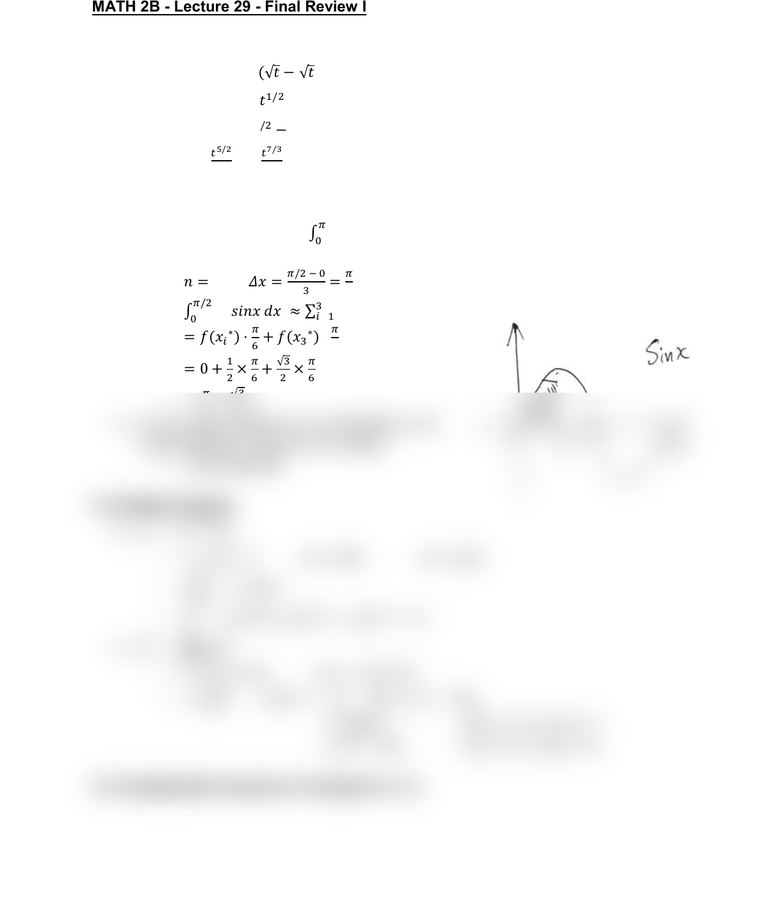Class Notes (1,100,000)
US (460,000)
UC-Irvine (10,000)
MATH (1,000)
MATH 2B (600)
Lecture 29

# MATH 2B Lecture Notes - Lecture 29: Riemann Sum, AntiderivativePremium

Department
Mathematics
Course Code
MATH 2B
Professor
ERJAEE, G.
Lecture
29

This preview shows half of the first page. to view the full 2 pages of the document.03/13/19
MATH 2B - Lecture 29 - Final Review I
4.9 Antiderivative
Compute 
:
 
 
 


5.1 Riemann Sum Rectangle Approximation
Approximate the integral 
 using a Riemann sum with three
rectangles and left endpoints
 




Is your approximation an overestimate or an
Underestimate
5.2 Definite Integral

 








 



 
 
5.3 Fundamental Theorem of Calculus (F.T.C)